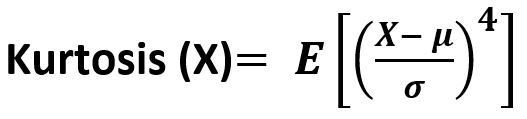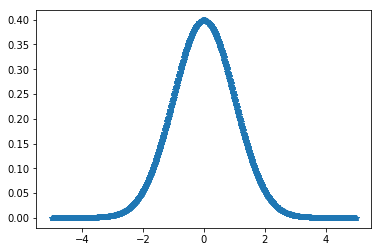Skip to content
Related Articles
scipy stats.kurtosis() function | Python
• Last Updated : 11 Feb, 2019

`scipy.stats.kurtosis(array, axis=0, fisher=True, bias=True)` function calculates the kurtosis (Fisher or Pearson) of a data set. It is the the fourth central moment divided by the square of the variance. It is a measure of the “tailedness” i.e. descriptor of shape of probability distribution of a real-valued random variable. In simple terms, one can say it is a measure of how heavy tail is compared to a normal distribution.

Its formula –Parameters :
array : Input array or object having the elements.
axis : Axis along which the kurtosis value is to be measured. By default axis = 0.
fisher : Bool; Fisher’s definition is used (normal 0.0) if True; else Pearson’s definition is used (normal 3.0) if set to False.
bias : Bool; calculations are corrected for statistical bias, if set to False.

Returns : Kurtosis value of the normal distribution for the data set.

Code #1:

 `# Graph using numpy.linspace() ``# finding kurtosis`` ` `from` `scipy.stats ``import` `kurtosis``import` `numpy as np ``import` `pylab as p `` ` `x1 ``=` `np.linspace( ``-``5``, ``5``, ``1000` `)``y1 ``=` `1.``/``(np.sqrt(``2.``*``np.pi)) ``*` `np.exp( ``-``.``5``*``(x1)``*``*``2`  `)`` ` `p.plot(x1, y1, ``'*'``)`` ` ` ` `print``( ``'\nKurtosis for normal distribution :'``, kurtosis(y1))`` ` `print``( ``'\nKurtosis for normal distribution :'``, ``      ``kurtosis(y1, fisher ``=` `False``))`` ` `print``( ``'\nKurtosis for normal distribution :'``, ``      ``kurtosis(y1, fisher ``=` `True``))`

Output :

```Kurtosis for normal distribution : -0.3073930877422071

Kurtosis for normal distribution : 2.692606912257793

Kurtosis for normal distribution : -0.3073930877422071
```

Attention geek! Strengthen your foundations with the Python Programming Foundation Course and learn the basics.

To begin with, your interview preparations Enhance your Data Structures concepts with the Python DS Course. And to begin with your Machine Learning Journey, join the Machine Learning – Basic Level Course

My Personal Notes arrow_drop_up